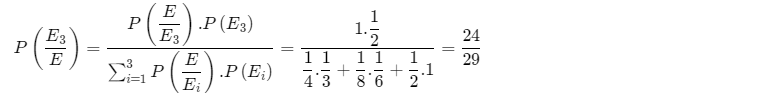Click to Chat

1800-1023-196

+91-120-4616500

CART 0

• 0

MY CART (5)

Use Coupon: CART20 and get 20% off on all online Study Material

ITEM
DETAILS
MRP
DISCOUNT
FINAL PRICE
Total Price: Rs.

There are no items in this cart.
Continue Shopping
```
In a test an examinee either guesses or copies or knows that answer to a multiple choice question which has 4 choices. The probability that he makes a guess is 1/3 and the probability that he copies is 1/6. The probability that his answer is correct, given the copied it, is 1/8. Find the probability that he knew the answer to the question, given that he answered it correctly.

```
11 years ago

```							 P(g) = probability of guessing = 1/3
P(c) = probability of copying = 1/6
P(k) = probability of knowing = 1 - 1/3 - 1/6 = 1/2
(Since the three-event g, c and k are mutually exclusive and exhaustive)
P(w) = probability that answer is correct
P(k/w)=(P(w/k).P(k))/(P(w/c)P(c)+P(w/k)P(k)+P(w/g)P(g))                (using Baye's theorem)
= (1×1/2)/((1/8,1/6)+(1×1/2)+(1/4×1/3) )=24/29

```
11 years ago
```							P(w/c) can't be less than 1/4..As there are 4 choices to the multiple choice question and even if he copies from someone else's the minimum probability of getting a correct choice is greater than or equal to 1/4.
```
one year agoYash Chourasiya
246 Points
```							Dear StudentLet define the following events:E1​:Examine guesses the answer to the question.E2​:Examine copies the answer to the question.E3​:Examine know the answer to the question.E:answer is correctHereP(E1​) = 1/3, P(E2​) = 1/6​∴P(E3​) = 1−(1/3​+1/6​) = 1/2​Since the question is a multiple choice question with four choice so the probability that the answer is correctwhen is guessed isP(E/E1​) = 1/4​Also the probability that his answer is correct, given that he copied it is81​i.eP(E/E2​) = 1/8Moreover his answer is correct, given he knew the answer is a sure event, soP(E/E3​​) = 1Hence by Baye's theorem the required probability isI hope this answer will help you.Thanks & RegardsYash Chourasiya
```
2 months ago
Think You Can Provide A Better Answer ?

## Other Related Questions on Algebra

View all Questions »### Course Features

• 731 Video Lectures
• Revision Notes
• Previous Year Papers
• Mind Map
• Study Planner
• NCERT Solutions
• Discussion Forum
• Test paper with Video Solution### Course Features

• 101 Video Lectures
• Revision Notes
• Test paper with Video Solution
• Mind Map
• Study Planner
• NCERT Solutions
• Discussion Forum
• Previous Year Exam Questions